# 6. Induction

Proof by induction is a method of proving (countably) infinitely many propositions at a time. A proof by induction consists of a basis step and an induction step. In the basis step one proves the proposition for the simplest possible case. Then one proves that if the proposition holds for a specific case, then this implies that the proposition also holds for the next case. We can illustrate the procedure as an infinite line of domino bricks; the basis step corresponds to falling the first domino brick while the induction step corresponds to that if one brick falls, the next will also fall. It is not hard to realize that we in this case will fall all bricks by falling the first.

Axiom of induction: Let Pn be a proposition depending on the integer n. If

1) Basis step: P1 is true (proposition is true for n=1)
2) Induction steg: Pp implies Pp+1, that is, if the proposition is true for n=p then it must also be true for n=p+1

then the proposition is true for all integers n=1,2,3,…

Example 1: We note that 61=6, 62=36, 63=216, 64=1296, that is, the first 4 integer powers of 6 has the last digit 6. Let us show that this true for all positive integer powers 6n.
Basis step:
61=6 has last digit 6.

Induction step: We assume that the proposition is true for n=p, that is, 6phas last digit 6. This we can write asfor some integer k. If this assumption holds, we have for 6p+1 thatthat is, 6p+1 also has last digit 6.

Since we have shown that the proposition holds for the basis step n=1 as well as that the assumption that the proposition holds for n=p implies that it also holds for n=p+1, it follows from the principle of induction that the proposition is true for all integers n=1,2,3,…

Example 2: Show thatBasis step: We have that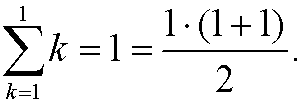Induction step: We assume that the formula is correct for n=p, that isWe want to show that it then also must hold thatWe have thatSince we have shown that the proposition holds for the basis step n=1 as well as that the assumption that the proposition holds for n=p implies that it also holds for n=p+1, it follows from the principle of induction that the proposition is true for all integers n=1,2,3,…

Example 3: Show thatis divisible by 7 for every n=1,2,3,…
Basis step: We have thatis divisible by 7.
Induction step: We assume thatis divisible by 7, that is, thatfor some integer k. Thenis also divisible by 7.
Since we have shown that the proposition holds for the basis step n=1 as well as that the assumption that the proposition holds for n=p implies that it also holds for n=p+1, it follows from the principle of induction that the proposition is true for all integers n=1,2,3,…

Example 4: Show that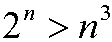for n=10,11,12,…
Basis step:Induction step: Assume that the inequality holds for n=p, that is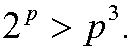We want to show that it then also must hold for n=p+1, that is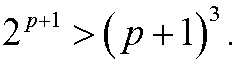We have thatsince p>9.

Since we have shown that the proposition holds for the basis step n=10 as well as that the assumption that the proposition holds for n=p implies that it also holds for n=p+1, it follows from the principle of induction that the proposition is true for all integers n=10,11,12,…

Example 5: Show that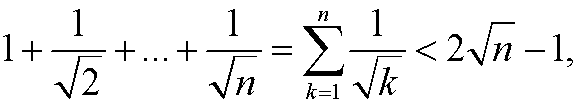for n=2,3,4,…
Basis step:Induction step: Assume that the inequality holds for n=p, that isWe want to show that it then also must hold for n=p+1, that isWe have according to the induction assumption thatIf we could have shown that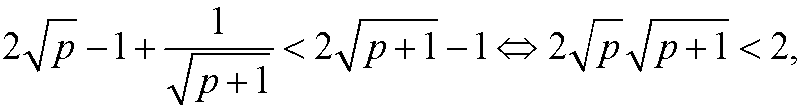we would have been finished. However,Since we have shown that the proposition holds for the basis step n=2 as well as that the assumption that the proposition holds for n=p implies that it also holds for n=p+1, it follows from the principle of induction that the proposition is true for all integers n=2,3,4,…

Example 6: Show that the recurrence equationhas the solutionBasis step:Induction step: Assume that the formula is correct for n=p, that isThen we have thatthat is, the formula is correct also for n=p+1. Since we have shown that the proposition holds for the basis step n=0 as well as that the assumption that the proposition holds for n=p implies that it also holds for n=p+1, it follows from the principle of induction that the proposition is true for all integers n=0,1,2,3,…

Example 7: Fibonacci‘s sequence {an}={1,1,2,3,5,8,13,21,…} is defined recursively byShow that the fibonacci numbersfor n=1,2,3…
Basis step: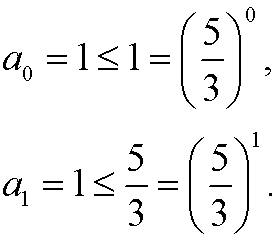Induction step: Assume that the inequality holds for n=p and for n=p-1, that is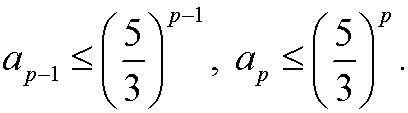We want to show that it also must hold for n=p+1, that is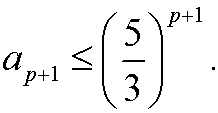We have according to the induction assumption thatSince we have shown that the proposition holds for the basis step n=0,n=1as well as that the assumption that the proposition holds for n=p-1 and n=pimplies that it also holds for n=p+1, it follows from the principle of induction that the proposition is true for all integers n=1,2,3,…